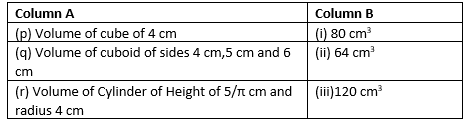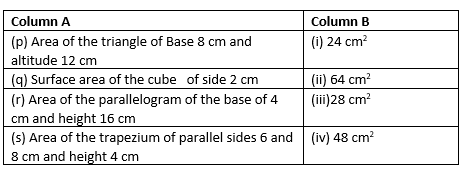# Mensuration class 8 worksheets

In this page we have Mensuration class 8 worksheets Chapter 11 . Hope you like them and do not forget to like , social share and comment at the end of the page.

Question 1
Find the area of a trapezium whose parallel sides are 12 cm and 20 cm and the distance between them is 15 cm.

Question 2
Find the area of a trapezium whose parallel sides are 38.7 cm and 22.3 cm, and the distance between them is 18 cm.

Question 3
The area of a trapezium is 1440 cm2. If the lengths of its parallel sides are 54.6 cm and 35.4 cm, find the distance between them.

Question 4
The area of a trapezium is 1586 cm2 and the distance between its parallel sides is 26 cm. If one of the parallel sides is 84 cm, find the other.

Question 5
The area of a trapezium is 384cm2. Its parallel sides are in the ratio 2: 6 and the perpendicular distance between them is 12 cm. Find the length of each of the parallel sides.

Question 6
Mitesh wants to buy a trapezium shaped field. Its side along the river is parallel to and twice the side along the road. If the area of this field is 10500 m2 and the perpendicular distance between the two parallel sides is 100 m, find the length of the side along the river.

Question 7
The area of a trapezium is 180 cm2 and its height is 9 cm. If one of the parallel sides is longer than the other by 6 cm, find the two parallel sides.

Question 8
The parallel sides of a trapezium are 20 cm and 10 cm. Its nonparallel sides are both equal, each being 13 cm. Find the area of the trapezium.

## True and False

Question 9
(i) Amount of region occupied by a solid is called its surface area
(ii)The areas of any two faces of a cube are equal.
(iii)The areas of two oppossite faces of a cuboid are equal.
(iv) 2.5 litres is equal to 0.0025 cubic meters
(v) Ratio of area of a circle to the area of a square whose side equals radius of circle is 1 : π.

## Fill in the blanks

Question 10
(i) Curved surface area of a cylinder of radius 2b and height 2a is _______.
(ii) Volume of a cylinder with radius p and height q is __________.
(iii) 6.55m2 = ______cm2
(iv)The volume of a cylinder becomes __________ the original volume if its radius becomes doubles of the original radius and height becomes half of its original values
(v)The surface area of a cylinder which exactly fits in a cube of side b is____

## Match the column

Question 11Question 12## Summary

This mensuration class 8 worksheets is prepared keeping in mind the latest syllabus of CBSE . This has been designed in a way to improve the academic performance of the students. If you find mistakes , please do provide the feedback on the mail.